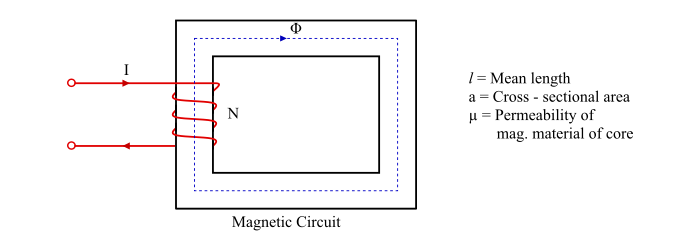# Magnetic Reluctance: Definition, Formula & Examples

The magnetic reluctance (S) is defined as the opposition offered by the magnetic circuit to the magnetic flux (Φ

## Magnetic Reluctance Formula### Case 1 – When physical dimensions of the magnetic circuit are known

The reluctance of a magnetic circuit depends upon its length (l), cross-sectional area (a) and permeability (μ) of the material. Thus, for a magnetic circuit (as shown in the figure),

• The reluctance is directly proportional to the mean length of the magnetic circuit, i.e.

$$\mathrm{Magnetic\:reluctance,S\varpropto\:l\:\:\:\:....(1)}$$

• The reluctance is inversely proportional to the cross-sectional area of the mag. circuit.

$$\mathrm{Magnetic\:reluctance,S\varpropto\:\frac{1}{a}\:\:\:\:....(2)}$$

• The reluctance also depends upon the nature of material that makes up the mag. circuit.

eqs. (1) & (2), it can be written,

$$\mathrm{Magnetic\:reluctance,S\varpropto\:\frac{1}{a}}$$

$$\mathrm{Magnetic\:reluctance,S\varpropto\:\frac{1}{\mu_{0}\mu_{r}a}\:\:\:\:....(3)}$$

### Case 2 – When mmf and magnetic flux in the circuit are known

If mmf (NI) produced and magnetic flux (Φ) flowing in the magnetic circuit are the known quantities, then the reluctance of the magnetic circuit can be given as,

$$\mathrm{Reluctance,S=\frac{mmf(NI)}{Magnetic\:flux(\phi)}\:\:\:\:...(4)}$$

## Unit of Magnetic Reluctance

Since, the magnetic reluctance is given by

$$\mathrm{S=\frac{mmf(NI)}{Magnetic\:flux(\phi)}=\frac{Ampere\times\:Turn}{Weber}=AT/Wb}$$

Therefore, the magnetic reluctance is measured in AT/Wb.

Note – The reluctance of magnetic materials (like iron, steel etc.) is low due to their high relative permeability whereas the non-magnetic materials (such as air, wood, copper etc.) have high reluctance due to low relative permeability.

## Numerical Example (1)

A magnetic circuit consists of an iron ring uniformly wounded with 1750 turns of insulated wire and a magnetising current of 1 A produces a magnetic flux of 1mWb. Neglect the effect of magnetic leakage. Determine the reluctance of magnetic circuit.

Solution

Reluctance,

$$\mathrm{S=\frac{Total\:mmf(NI)}{Magnetic\:flux()\phi}}$$

$$\mathrm{=\frac{1750\times\:1}{1\times\:10^{-3}}}$$

$$\mathrm{=1.75\times\:10^{6}\:\:\:AT/Wb}$$

## Numerical Example (2)

The magnetic circuit is built up of iron of square cross section 2 cm sides and the mean length of the magnetic circuit is 54 cm. The relative permeability of material that makes up the magnetic circuit is 1200. Find the magnetic reluctance of the given magnetic circuit.

Solution

Area of cross-section,

$$\mathrm{a=2\times\:2=4\:cm^{2}=4\times\:10^{-4}\:m^{2}}$$

Reluctance,

$$\mathrm{S=\frac{1}{\mu_{0}\mu_{r}a}=\frac{54\times\:10^{-2}}{(4\pi\times\:10^{-7})\times\:(1200)\times\:(4\times\:10^{-4})}}$$

$$\mathrm{\Rightarrow\:S=895700.64\:AT/Wb}$$